#### 4.10Spatial Transformations

This section gives the API for applying spatial transforms to a flomap, such as rotations, warps, morphs, and lens distortion effects.

To use the provided transforms, apply a function like flomap-flip-horizontal directly, or apply something like a flomap-rotate-transform to a flomap using flomap-transform.

To make your own transforms, compose existing ones with flomap-transform-compose, or construct a value of type Flomap-Transform directly:
 (: my-awesome-transform Flomap-Transform) (define (my-awesome-transform w h) (make-flomap-2d-mapping fun inv))
Here, fun is a mapping from input coordinates to output coordinates and inv is its inverse.

Contents:

##### 4.10.1Provided Transformations

 procedure fm : flomap
 procedure fm : flomap
 procedure fm : flomap
 procedure fm : flomap
 procedure fm : flomap
Some standard image transforms. These are lossless, in that repeated applications do not degrade (blur or alias) the image.

Examples:
> (require pict)
 > (define text-fm (flomap-trim (bitmap->flomap (pict->bitmap (vc-append (text "We CLAIM the" '(bold) 25) (text "PRIVILEGE" '(bold) 25))))))
> (flomap->bitmap text-fm)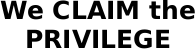> (flomap->bitmap (flomap-flip-horizontal text-fm))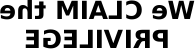> (flomap->bitmap (flomap-flip-vertical text-fm))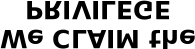> (flomap->bitmap (flomap-transpose text-fm))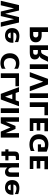> (flomap->bitmap (flomap-cw-rotate text-fm))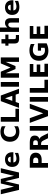> (flomap->bitmap (flomap-ccw-rotate text-fm))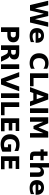> (equal? (flomap-cw-rotate fm) (flomap-flip-vertical (flomap-transpose fm)))

#t

 > (equal? (flomap-ccw-rotate fm) (flomap-flip-horizontal (flomap-transpose fm)))

#t

 procedure(flomap-rotate fm θ) → flomap fm : flomap θ : Real
Returns a flomap rotated by θ radians counterclockwise. Equivalent to (flomap-transform fm (flomap-rotate-transform θ)).

Example:
 > (flomap->bitmap (flomap-rotate text-fm (* 1/4 pi)))procedure θ : Real
Returns a flomap transform that rotates a flomap θ radians counterclockwise around its (Real-valued) center.

Use flomap-rotate-transform if you need to know the bounds of the rotated flomap or need to compose a rotation with another transform using flomap-transform-compose.

Examples:
 > (flomap-transform-bounds (flomap-rotate-transform (* 1/4 pi)) 100 100)
 -21 -21 121 121
 > (flomap->bitmap (flomap-transform text-fm (flomap-rotate-transform (* 1/4 pi))))procedure θ : Real
Returns a flomap transform that “whirls” a flomap: rotates it counterclockwise θ radians in the center, and rotates less with more distance from the center.

This transform does not alter the size of its input.

Example:
 > (flomap->bitmap (flomap-transform text-fm (flomap-whirl-transform pi)))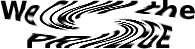procedure α : Real
Returns a flomap transform that simulates “fisheye” lens distortion with an α diagonal angle of view. Equivalent to
 (flomap-projection-transform (equal-area-projection α) (perspective-projection α) #f)

Example:
 > (flomap->bitmap (flomap-transform text-fm (flomap-fisheye-transform (* 2/3 pi))))procedure(flomap-scale-transform x-scale [y-scale]) → Flomap-Transform x-scale : Real y-scale : Real = x-scale
Returns a flomap transform that scales flomaps by x-scale horizontally and y-scale vertically.

You should generally prefer to use flomap-scale, which is faster and correctly reduces resolution before downsampling to avoid aliasing. This is provided for composition with other transforms using flomap-transform-compose.

 value
A flomap transform that does nothing. See flomap-transform-compose for an example of using flomap-id-transform as the initial value for a fold.

##### 4.10.2General Transformations

procedure

(flomap-transform fm t)  flomap

fm : flomap
t : Flomap-Transform
 (flomap-transform fm t x-start y-start x-end y-end) → flomap
fm : flomap
t : Flomap-Transform
x-start : Integer
y-start : Integer
x-end : Integer
y-end : Integer
Applies spatial transform t to fm.

The rectangle x-start y-start x-end y-end is with respect to the fm’s transformed coordinates. If given, points in fm are transformed only if their transformed coordinates are within that rectangle. If not given, flomap-transform uses the rectangle returned by (flomap-transform-bounds t w h), where w and h are the size of fm.

This transform doubles a flomap’s size:
 > (define (double-transform w h) (make-flomap-2d-mapping (λ (x y) (values (* x 2) (* y 2))) (λ (x y) (values (/ x 2) (/ y 2)))))
 > (flomap->bitmap (flomap-transform text-fm double-transform))Transforms can use the width and height arguments w h however they wish; for example, double-transform ignores them, and flomap-rotate-transform uses them to calculate the center coordinate.

The flomap-rotate function usually increases the size of a flomap to fit its corners in the result. To rotate in a way that does not change the size—i.e. to do an in-place rotation—use 0 0 w h as the transformed rectangle:
 > (define (flomap-in-place-rotate fm θ) (define-values (w h) (flomap-size fm)) (flomap-transform fm (flomap-rotate-transform θ) 0 0 w h))
Using it on text-fm with a purple background:
> (define-values (text-fm-w text-fm-h) (flomap-size text-fm))
 > (define purple-text-fm (flomap-lt-superimpose (make-flomap* text-fm-w text-fm-h #(1 1/2 0 1)) text-fm))
> (flomap->bitmap purple-text-fm)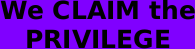> (flomap->bitmap (flomap-in-place-rotate purple-text-fm (* 1/8 pi)))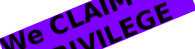See flomap-projection-transform for another example of using flomap-transform’s rectangle arguments, to manually crop a lens projection.

Alternatively, we could define a new transform-producing function flomap-in-place-rotate-transform that never transforms points outside of the orginal flomap:
 > (define ((flomap-in-place-rotate-transform θ) w h) (match-define (flomap-2d-mapping fun inv _) ((flomap-rotate-transform θ) w h)) (make-flomap-2d-mapping (λ (x y) (let-values ([(x y)  (fun x y)]) (values (if (<= 0 x w) x +nan.0) (if (<= 0 y h) y +nan.0)))) inv))
 > (flomap->bitmap (flomap-transform purple-text-fm (flomap-in-place-rotate-transform (* 1/8 pi))))To transform fm, flomap-transform uses only the inv field of (t w h). Every point new-x new-y in the transformed bounds is given the components returned by
 (let-values ([(old-x old-y)  (inv new-x new-y)]) (flomap-bilinear-ref* fm old-x old-y))
The forward mapping fun is used by flomap-transform-bounds.

 syntax

A value of type Flomap-Transform receives the width and height of a flomap to operate on, and returns a flomap-2d-mapping on the coordinates of flomaps of that size.

 struct(struct flomap-2d-mapping (fun inv bounded-by)) fun : (Float Float -> (values Float Float)) inv : (Float Float -> (values Float Float)) bounded-by : (U 'id 'corners 'edges 'all)
Represents an invertible mapping from Real×Real to Real×Real, or from real-valued flomap coordinates to real-valued flomap coordinates. See flomap-transform for examples. See Conceptual Model for the meaning of real-valued flomap coordinates.

The forward mapping fun is used to determine the bounds of a transformed flomap. (See flomap-transform-bounds for details.) The inverse mapping inv is used to actually transform the flomap. (See flomap-transform for details.)

The symbol bounded-by tells flomap-transform-bounds how to transform bounds. In order of efficiency:
• 'id: Do not transform bounds. Use this for in-place transforms such as flomap-whirl-transform.

• 'corners: Return the smallest rectangle containing only the transformed corners. Use this for linear and affine transforms (such as flomap-rotate-transform or a skew transform), transforms that do not produce extreme points, and others for which it can be proved (or at least empirically demonstrated) that the rectangle containing the transformed corners contains all the transformed points.

• 'edges: Return the smallest rectangle containing only the transformed left, top, right, and bottom edges. Use this for transforms that are almost-everywhere continuous and invertible—which describes most interesting transforms.

• 'all: Return the smallest rectangle containing all the transformed points. Use this for transforms that produce overlaps and other non-invertible results.

For good performance, define instances of flomap-2d-mapping and functions that return them (e.g. instances of Flomap-Transform), in Typed Racket. Defining them in untyped Racket makes every application of fun and inv contract-checked when used in typed code, such as the implementation of flomap-transform. (In the worst case, flomap-transform applies fun to every pair of coordinates in the input flomap. It always applies inv to every pair of coordinates in the output flomap.)

 procedure(make-flomap-2d-mapping fun inv [bounded-by]) → flomap-2d-mapping fun : (Float Float -> (values Real Real)) inv : (Float Float -> (values Real Real)) bounded-by : (U 'id 'corners 'edges 'all) = 'edges
A more permissive, more convenient constructor for flomap-2d-mapping.

 procedure t2 : Flomap-Transform t1 : Flomap-Transform
Composes two flomap transforms. Applying the result of (flomap-transform-compose t2 t1) is the same as applying t1 and then t2, except:
• The points are transformed only once, meaning their component values are estimated only once, so the result is less degraded (blurry or aliased).

• The bounds are generally tighter.

The following example “whirls” text-fm clockwise 360 degrees and back. This is first done by applying the two transforms separately, and secondly by applying a composition of them.
 > (let* ([text-fm  (flomap-transform text-fm (flomap-whirl-transform (* 2 pi)))] [text-fm  (flomap-transform text-fm (flomap-whirl-transform (* -2 pi)))]) (flomap->bitmap text-fm))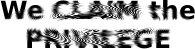> (flomap->bitmap (flomap-transform text-fm (flomap-transform-compose (flomap-whirl-transform (* -2 pi)) (flomap-whirl-transform (* 2 pi)))))Notice the heavy aliasing (a “Moiré pattern”) in the first result is not in the second.

In the next example, notice that rotating multiple times blurs the result and pads it with transparent points, but that applying composed rotation transforms doesn’t:
 > (let* ([text-fm  (flomap-rotate text-fm (* 1/8 pi))] [text-fm  (flomap-rotate text-fm (* 1/8 pi))] [text-fm  (flomap-rotate text-fm (* 1/8 pi))] [text-fm  (flomap-rotate text-fm (* 1/8 pi))]) (flomap->bitmap text-fm))> (define rotate-pi/2 (for/fold ([t flomap-id-transform]) ([_  (in-range 4)]) (flomap-transform-compose (flomap-rotate-transform (* 1/8 pi)) t)))
> (flomap->bitmap (flomap-transform text-fm rotate-pi/2))How the bounds for the composed transform are calculated depends on how they would have been calculated for t1 and t2. Suppose b1 is the bounds rule for (t1 w h) and b2 is the bounds rule for (t2 w h). Then the bounds rule b for (flomap-transform-compose t2 t1) is determined by the following rules, applied in order:
• If either b1 = 'all or b2 = 'all, then b = 'all.

• If either b1 = 'edges or b2 = 'edges, then b = 'edges.

• If either b1 = 'corners or b2 = 'corners, then b = 'corners.

• Otherwise, b1 = b2 = 'id, so b = 'id.

See flomap-2d-mapping for details on how b affects bounds calculation.

procedure

(flomap-transform-bounds t w h)
 Integer Integer Integer Integer
t : Flomap-Transform
w : Integer
h : Integer
Returns the rectangle that would contain a w×h flomap after transform by t.

How the rectangle is determined depends on the bounded-by field of (t w h). See flomap-2d-mapping for details.

See flomap-rotate-transform and flomap-projection-transform for examples.

##### 4.10.3Lens Projection and Correction

The following API demonstrates a parameterized family of spatial transforms. It also provides a physically grounded generalization of the flomap transforms returned by flomap-fisheye-transform.

procedure

 (flomap-projection-transform to-proj from-proj crop?) → Flomap-Transform
to-proj : Projection
from-proj : Projection
crop? : Boolean
Returns a flomap transform that corrects for or simulates lens distortion.

To correct for lens distortion in a flomap:
• Find a projection from-proj that models the actual lens.

• Find a projection to-proj that models the desired (but fictional) lens.

• Apply (flomap-projection-transform to-proj from-proj) to the flomap.

This photo is in the public domain. In the following example, a photo of the State of the Union address was taken using an “equal area” (or “equisolid angle”) fisheye lens with a 180-degree diagonal angle of view:
 > (flomap->bitmap state-of-the-union-fm)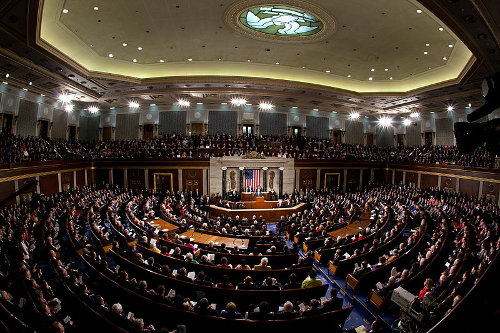We would like it to have been taken with a perfect “rectilinear” (or “perspective projection”) lens with a 120-degree diagonal angle of view. Following the steps above, we apply a projection transform using (equal-area-projection (degrees->radians 180)) for from-proj and (perspective-projection (degrees->radians 120)) for to-proj:
 > (flomap->bitmap (flomap-transform state-of-the-union-fm (flomap-projection-transform (perspective-projection (degrees->radians 120)) (equal-area-projection (degrees->radians 180)))))Notice that the straight geometry in the House chamber (especially the trim around the ceiling) is represented by straight edges in the corrected photo.

When crop? is #t, the output flomap is no larger than the input flomap. When crop? is #f, the output flomap is large enough to contain the entire transformed flomap. An uncropped result can be quite large, especially with angles of view at or near 180 degrees.
 > (define rectangle-fm (draw-flomap (λ (fm-dc) (send fm-dc set-pen "black" 4 'dot) (send fm-dc set-brush "yellow" 'solid) (send fm-dc set-alpha 1/2) (send fm-dc draw-rectangle 0 0 32 32)) 32 32))
> (flomap->bitmap rectangle-fm)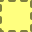> (flomap-transform-bounds (flomap-projection-transform (perspective-projection (degrees->radians 90)) (equal-area-projection (degrees->radians 180)) #f) 32 32)
 -56481829139474512 -56481829139474520 56481829139474552 56481829139474552
 > (flomap->bitmap (flomap-transform rectangle-fm (flomap-projection-transform (perspective-projection (degrees->radians 90)) (orthographic-projection (degrees->radians 160)) #f)))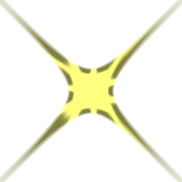To crop manually, apply flomap-transform to explicit rectangle arguments:
 > (flomap->bitmap (flomap-transform rectangle-fm (flomap-projection-transform (perspective-projection (degrees->radians 90)) (orthographic-projection (degrees->radians 160)) #f) -10 -10 42 42))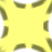procedure α : Real
 procedure α : Real
 procedure α : Real
 procedure α : Real
 procedure α : Real
Given a diagonal angle of view α, these all return a projection modeling some kind of camera lens. See Fisheye Lens for the defining formulas.

 syntax
Equivalent to (Float -> projection-mapping).

A value of type Projection receives the diagonal size of a flomap to operate on, and returns a projection-mapping instance. The provided projections (such as perspective-projection) use a closed-over diagonal angle of view α and the diagonal size to calculate the focal length.

 struct(struct projection-mapping (fun inv)) fun : (Float -> Float) inv : (Float -> Float)
Represents an invertible function from a point’s angle ρ from the optical axis, to the distance r to the center of a photo, in flomap coordinates.

For example, given a diagonal angle of view α and the diagonal size d of a flomap, the perspective-projection function calculates the focal length f:

(define f (/ d 2.0 (tan (* 0.5 α))))

It then constructs the projection mapping as
 (projection-mapping (λ (ρ) (* (tan ρ) f)) (λ (r) (atan (/ r f))))
See Fisheye Lens for details.